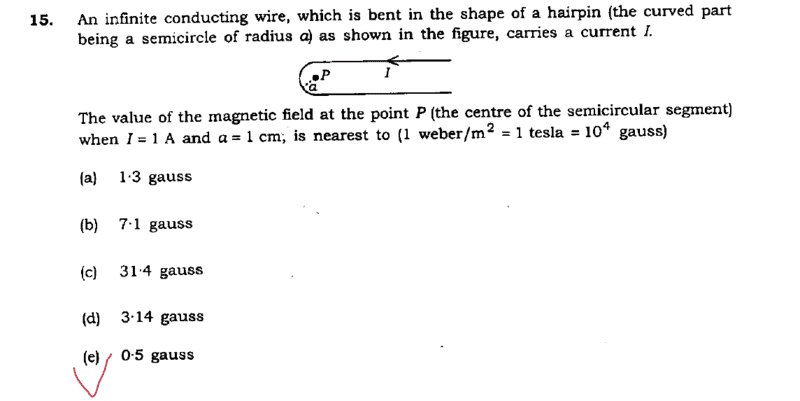# B on center of 2 infinite wires with semi - circular end

## Homework Statement## The Attempt at a Solution

Magnetic field due to both semi - infinite straight wires on P = Magnetic field due to infinite straight wire on P = ## \frac { \mu_0 I } { 2 \pi a } = 2 * 10 ^{-5} ~wb/m^2 ##

Magnetic field due to semi – circular wire on P = ## \frac { \mu_0 I } { 4a} = 3.14 * 10 ^{-5} ~wb/m^2 ##

So, total magnetic field magnitude on P is = 0.514 gauss.

Is this correct?

#### Attachments

•Delta2